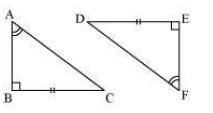# Class-7 Maths chapter-7 Congruence of Triangles Exercise-7.1

Question 1:
Complete the following statements:
(a) Two line segments are congruent if __________.
(b) Among two congruent angles, one has a measure of 70°; the measure of the other angle is __________.
(c) When we write ∠A = ∠ B, we actually mean __________.
(a) They have the same length
(b) 70°
(c) m ∠A = m ∠B

Question 2:
Give any two real-life examples for congruent shapes.
(i) Sheets of same letter pad
(ii) Biscuits in the same packet

Question 3:
If /ABC ≅ /FED under the correspondence ABC ↔ FED, write all the Corresponding congruent parts of the triangles.
If these triangles are congruent, then the corresponding angles and sides will be equal to each other.
∠A ↔ ∠F
∠B ↔ ∠E
∠C ↔ ∠D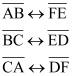Question 4:
If /DEF ≅ /BCA, write the part(s) of /BCA that correspond to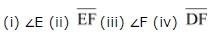(i) ∠C

### Exercise-7.2

Question 1:
Which congruence criterion do you use in the following?
(a) Given: AC = DF
AB = DE
BC = EF
So, /ABC ≅ /DEF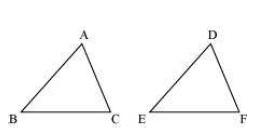(b) Given: ZX = RP
RQ = ZY
∠PRQ = ∠XZY
So, /PQR ≅ /XYZ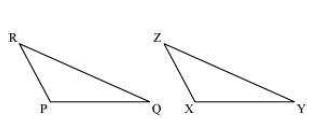(c) Given: ∠MLN = ∠FGH
∠NML = ∠GFH
ML = FG
So, /LMN ≅ /GFH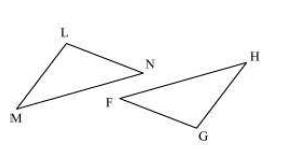(d) Given: EB = DB
AE = BC
∠A = ∠C = 90°
So, /ABE ≅ /CDB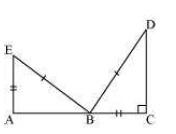(a) SSS, as the sides of /ABC are equal to the sides of /DEF.
(b) SAS, as two sides and the angle included between these sides of /PQR are equal to two sides and the angle included between these sides of /XYZ.
(c) ASA, as two angles and the side included between these angles of /LMN are equal to two angles and the side included between these angles of /GFH.
(d) RHS, as in the given two right-angled triangles, one side and the hypotenuse are respectively equal.

Question 2:
You want to show that /ART ≅ /PEN,
(a) If you have to use SSS criterion, then you need to show
(i) AR = (ii) RT = (iii) AT =
(b) If it is given that ∠T = ∠N and you are to use SAS criterion, you need to have
(i) RT = and (ii) PN =
(c) If it is given that AT = PN and you are to use ASA criterion, you need to have
(i) ? (ii) ?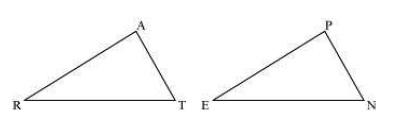(a)
(i) AR = PE
(ii) RT = EN
(iii) AT = PN
(b)
(i) RT = EN
(ii) PN = AT
(c)
(i) ∠ATR = ∠PNE
(ii) ∠RAT = ∠EPN

Question 3:
You have to show that /AMP ≅ AMQ.
In the following proof, supply the missing reasons.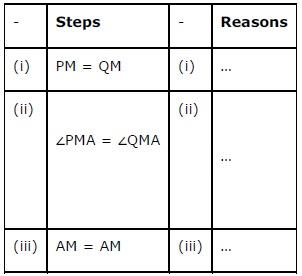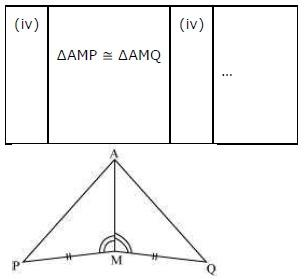(i) Given
(ii) Given
(iii) Common
(iv) SAS, as the two sides and the angle included between these sides of /AMP are equal to two sides and the angle included between these sides of /AMQ.

Question 4:
In /ABC, ∠A = 30°, ∠B = 40° and ∠C = 110°
In /PQR, ∠P = 30°, ∠Q = 40° and ∠R = 110°
A student says that /ABC ≅ /PQR by AAA congruence criterion. Is he justified? Why or why not?
No. This property represents that these triangles have their respective angles of equal measure. However, this gives no information about their sides. The sides of these triangles have a ratio somewhat different than 1:1. Therefore, AAA property does not prove the two triangles congruent.

Question 5:
In the figure, the two triangles are congruent.
The corresponding parts are marked. We can write /RAT ≅ ?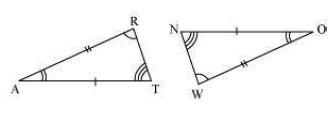It can be observed that,
∠RAT = ∠WON
∠ART = ∠OWN
AR = OW
Therefore, /RAT /WON, by ASA criterion.

Question 6:
Complete the congruence statement:
/BCA ≅?
/QRS ≅?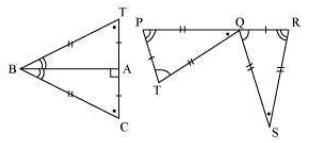Given that, BC = BT
TA = CA
BA is common.
Therefore, /BCA /BTA
Similarly, PQ = RS
TQ = QS
PT = RQ
Therefore, /QRS /TPQ

Question 7:
In a squared sheet, draw two triangles of equal areas such that
(i) The triangles are congruent.
(ii) The triangles are not congruent.
What can you say about their perimeters?
(i)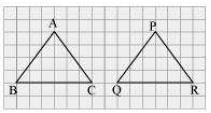Here, /ABC and /PQR have the same area and are congruent to each other also. Also, the perimeter of both the triangles will be the same.
(ii)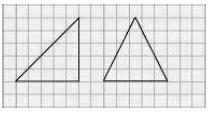Here, the two triangles have the same height and base. Thus, their areas are equal. However, these triangles are not congruent to each other. Also, the perimeter of both the triangles will not be the same.

Question 9:
If /ABC and /PQR are to be congruent, name one additional pair of corresponding parts. What criterion did you use?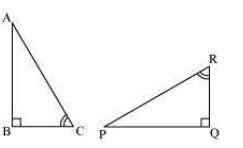BC = QR
/ABC /PQR (ASA criterion)

Question 10:
Explain, why
/ABC ≅ /FED